# Micro Binary Clock

Utilising a Microbit and a RTC to create a Binary clock with alarm option housed in a 3d printed box with resin inlay text.

IntermediateFull instructions provided24 hours499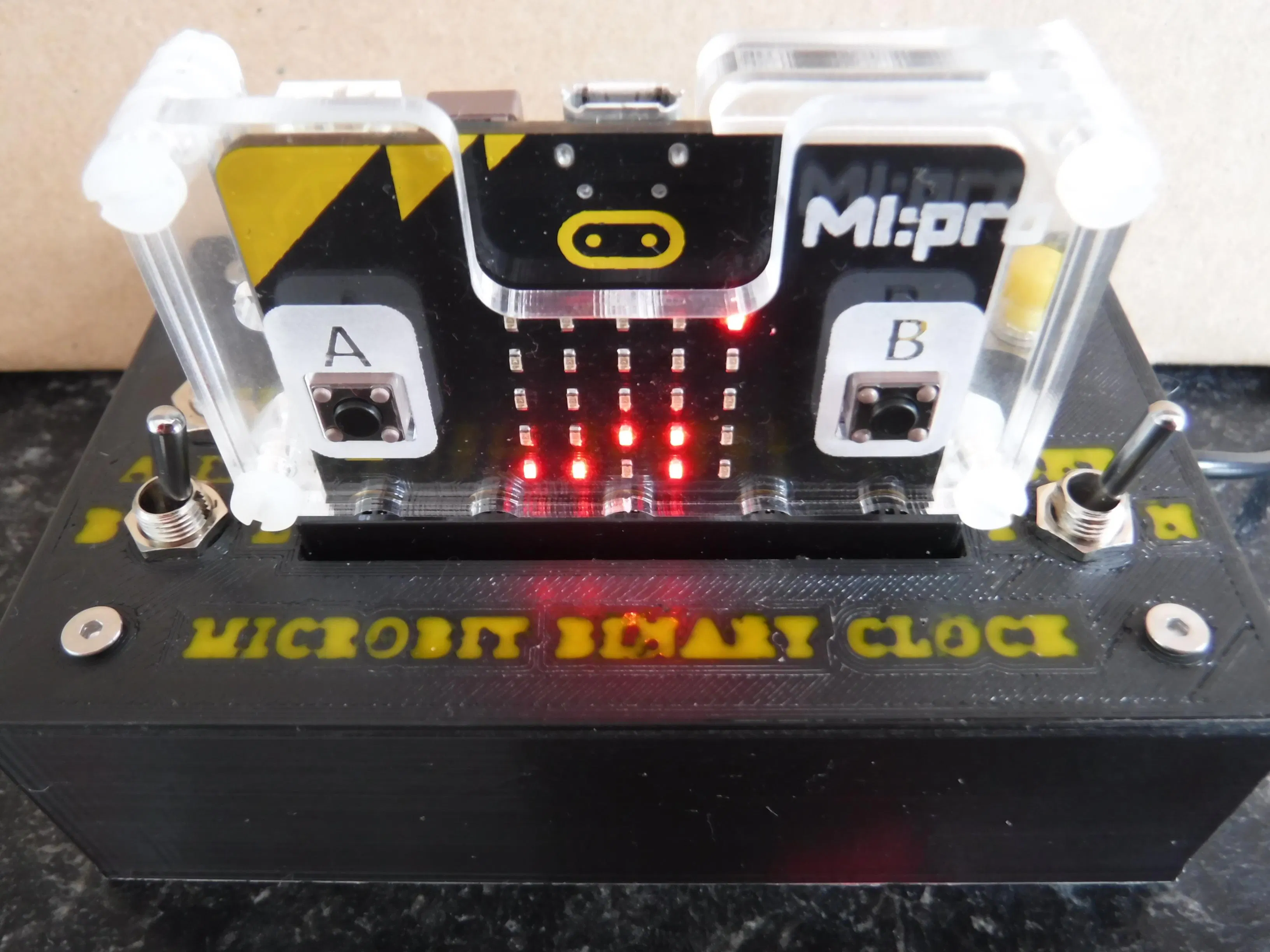## Things used in this project

### Hardware components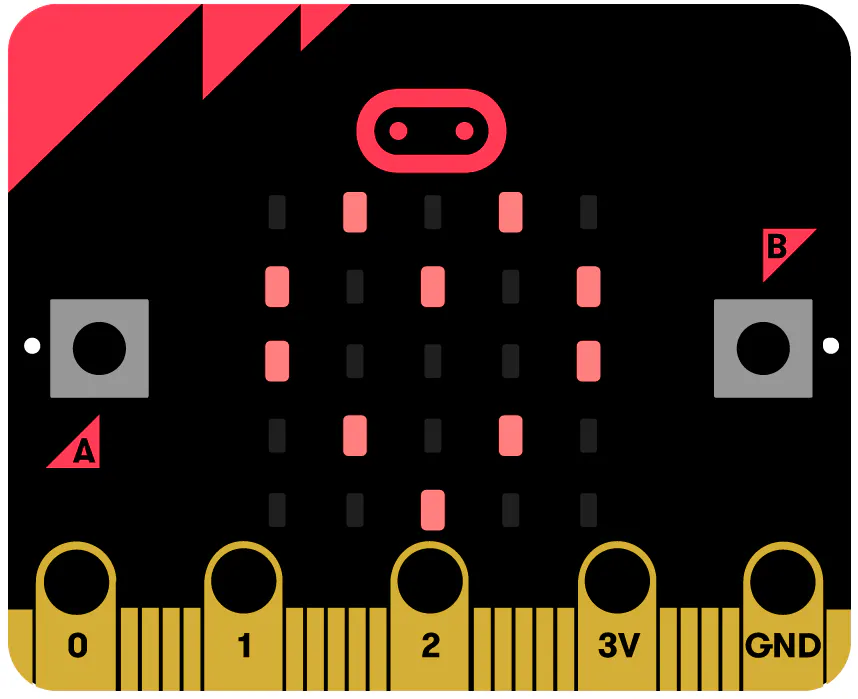BBC micro:bit board The project is compatible with both V1 and V2 boards.
×1
 Kitronik Klimate Environment Monitoring Board
×1
 SPDT (ON - ON) switch
×1
 SPDT (ON - OFF - ON) switch
×1
 SPDT (Normally Open), momentary switch
×2
 M3 (10+6mm), M/F standoffs with M3 nuts
×4
 M3 (8mm), screws
×8
 M3 nuts
×4
 piezo buzzer (no driver)
×1
 Jumper wire
×1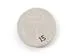Coin Cell Battery CR2032
×1

### Software apps and online servicesMicrosoft MakeCode
 Cura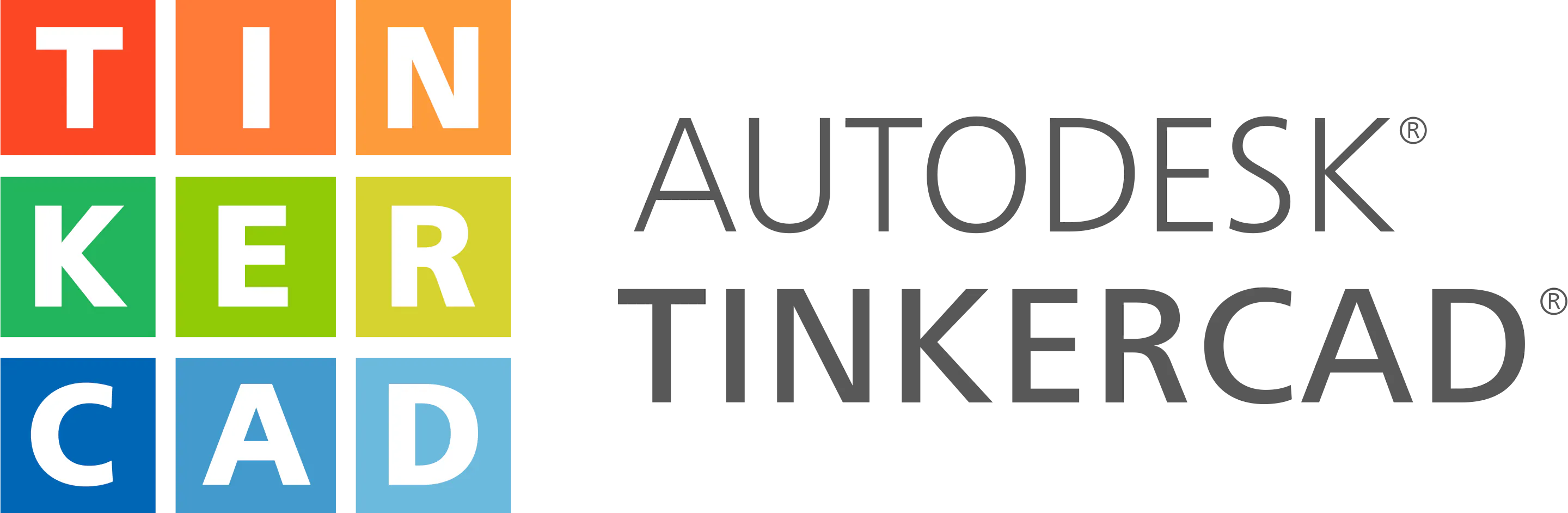### Hand tools and fabrication machines3D Printer (generic)

## Custom parts and enclosures

### Box base

Object file to enable 3d printing

### Box lid

Object file to enable 3d printing

## Schematics

### External connections

External inputs and output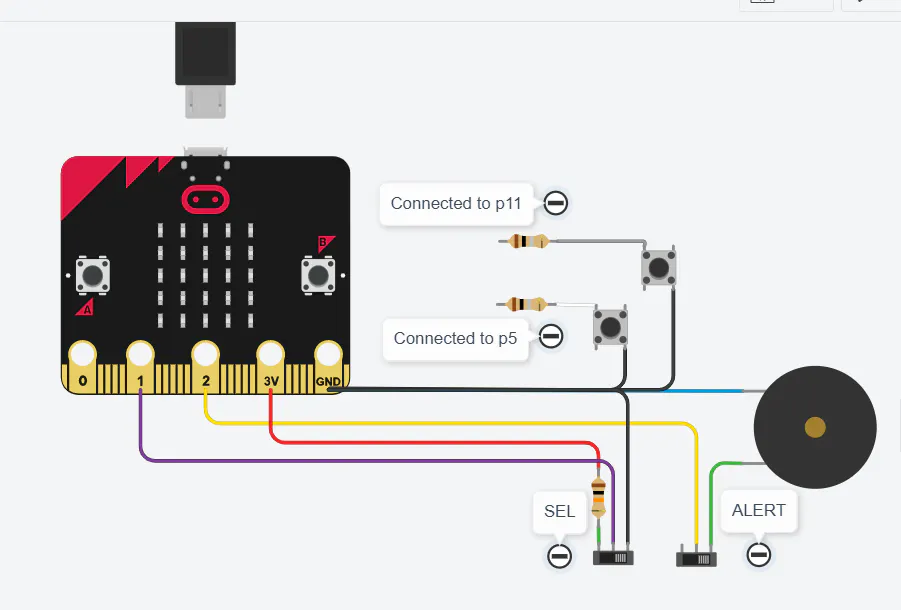## Code

### clk3.txt

Python
Microbit binary clock code
```def tick():
if tick_en == 1:
led.plot(4, 0)
basic.pause(1000)
led.unplot(4, 0)
basic.pause(1000)
showtm()
alarm_mode()
def dec2bin(value: number, col: number):
global list2, index4, index22
col = col
list2 = [0, 0, 0, 0]
index4 = 0
while index4 < len(list2):
if value > 1:
list2[index4] = value % 2
value = Math.idiv(value, 2)
else:
list2[index4] = value
break
index4 += 1
list2.reverse()
index22 = 0
while index22 < len(list2):
if list2[index22] == 1:
led.plot(col, index22 + 1)
else:
led.unplot(col, index22 + 1)
index22 += 1
def sound_alarm():
pins.analog_set_pitch_pin(AnalogPin.P2)
pins.analog_pitch(400, 1000)
# time column to set

def on_button_pressed_a():
global inc, tick_en, tm_hset, tm_mset, period, al_hset, al_mset
inc = 0
tick_en = 0
tm_hset = 0
tm_mset = 0
led.plot(period, 0)
if period > 0:
led.unplot(period - 1, 0)
if period > 3:
led.unplot(period, 0)
period = 0
tick_en = 1
tm_hset = tm_list * 10 + tm_list
tm_mset = tm_list * 10 + tm_list
if mode == 1:
kitronik_RTC.set_time(tm_hset, tm_mset, 0)
else:
al_hset = tm_hset
al_mset = tm_mset
else:
period += 1
input.on_button_pressed(Button.A, on_button_pressed_a)

def alarm_mode():
if alarm == 1:
for index in range(2):
led.plot(4, index + 3)
basic.pause(100)
for index2 in range(2):
led.unplot(4, index2 + 3)
basic.pause(100)
sound_alarm()
def rdtime():
global strval, alarm
if mode == 2:
alarm = 1
else:
alarm = 0
# mode

def on_button_pressed_ab():
global alarm, mode
alarm = 0
led.plot(4, mode + 1)
if mode > 0:
led.unplot(4, mode - 0)
else:
rdtime()
if mode > 1:
led.unplot(4, mode + 1)
mode = 0
led.plot(4, mode + 1)
else:
show_alarm()
mode += 1
input.on_button_pressed(Button.AB, on_button_pressed_ab)

# time column value

def on_button_pressed_b():
global tm_max, inc
if tick_en == 0:
# Maximum value per time column HHMM
tm_max = [2, 9, 5, 9]
inc += 1
if inc > tm_max[period - 1]:
inc = 0
tm_list[period - 1] = inc
dec2bin(inc, period - 1)
input.on_button_pressed(Button.B, on_button_pressed_b)

def show_alarm():
global index3, strval, strmid
index3 = 0
strval = "" + convert_to_text(al_hset) + convert_to_text(al_mset)
while index3 < len(strval):
strmid = strval.char_at(index3)
dec2bin(parse_float(strmid), index3)
index3 += 1
def showtm():
global index3, col2, strmid
index3 = 0
col2 = 0
rdtime()
while index3 < len(strval):
strmid = strval.char_at(index3)
if strmid != ":":
dec2bin(parse_float(strmid), col2)
col2 += 1
index3 += 1
strmid = ""
index3 = 0
tm_max: List[number] = []
al_mset = 0
al_hset = 0
tm_mset = 0
tm_hset = 0
index22 = 0
value2 = 0
index4 = 0
list2: List[number] = []
col2 = 0
alarm = 0
tm_list: List[number] = []
inc = 0
tick_en = 0
period = 0
mode = 0
strval = ""
strval = ""
mode = 0
period = 0
tick_en = 1
inc = 0
# Decimal time values per column
tm_list = [0, 0, 0, 0]
alarm = 0
basic.show_string("Bclk")
basic.show_string("<Sel")
basic.show_string(">Inc")

def on_forever():
tick()
basic.forever(on_forever)
```

## Credits

### C Forde

8 projects • 1 follower